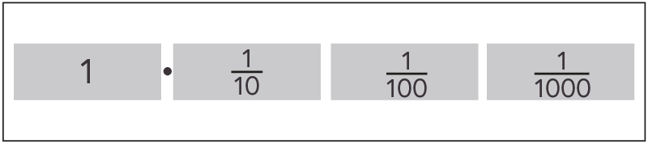## Want to keep learning?

This content is taken from the National STEM Learning Centre's online course, Maths Subject Knowledge: Fractions, Decimals, and Percentages. Join the course to learn more.
2.3

## National STEM Learning Centre

Skip to 0 minutes and 8 seconds PAULA KELLY: So let’s have a look, now, at a slightly more difficult example. If we’re trying to convert a fraction into a decimal– if we had, for example– let’s have 16/125.

Skip to 0 minutes and 23 seconds MICHAEL ANDERSON: OK, so these numbers look pretty daunting, but actually, it’s the exact same process. So if we look at the numerator, 16, it’s actually the denominator that we’re interested in– 125. We’re going to try and multiply this up to either a 10, 100, or most likely 1,000, or even bigger. So we’re going to try and think about 125 times tables. Now, actually, in order to get 1,000, we have to multiply 125 by 8.

Skip to 0 minutes and 55 seconds PAULA KELLY: And if we’re struggling with that, we could just do 125 times table.

Skip to 1 minute and 0 seconds MICHAEL ANDERSON: Yep. And this is obviously without a calculator as well. So yeah, we’re going to multiply by 8 to get 1,000 at the bottom. Now, we have to do the exact same to the top as well. So we’re going to take our 16 and multiply by 8 again, and 16 multiplied by 8– well, I can double it, double it, and double it again. And that will give me 128 over 1,000.

Skip to 1 minute and 24 seconds PAULA KELLY: OK, so we’ve seen, already, how we have this as a division. So 128 divided by 1,000– all of our numbers will go three places smaller.

Skip to 1 minute and 36 seconds MICHAEL ANDERSON: Yeah. And you can think about it in a different way as well. With our 128/1,000, you can think of it as 100 and 20 and 8. If you’ve got 100 over 1,000, that will simplify to 1 over 10. We’ve got 1/10. So I could put a 1 in the tens column, with a 20 part– well, 20 out of 1,000. I can divide both by 10, and I end up with 2 over 100– 2/100. And I can put the 2 in here. And that just leaves me with the 8, and I’ve got 8/1,000, so I can put 8 in the thousands column here. Now, 16 over 125 is less than 1.

Skip to 2 minutes and 14 seconds So in the units column, in the ones, I’m going to have a 0 there. So I end up with 0.128.

# Fractions as decimals: further example

In the previous step we saw how numbers expressed as fractions can be expressed as decimals. The examples used so far have been numbers which have one and two decimal places: tenths and hundredths. In this step we extend the method to include numbers which when expressed as a decimal have three decimal places: tenths, hundredths and thousandths.## Problem worksheet

Now complete question one from this week’s worksheet. As a reminder, problem worksheets are all linked in step 1.1, and at the start of each week.

## Teaching resource

This SMILE resource contains two packs of games, investigations, worksheets and practical activities supporting the teaching and learning of decimals, from reading decimals from a scale to multiplying two decimal numbers without a calculator.